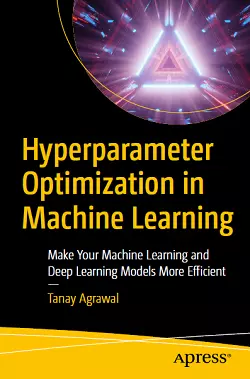# Hyperparameter Optimization in Machine Learning### eBook Details:

• Paperback: 188 pages
• Publisher: WOW! eBook (November 29, 2020)
• Language: English
• ISBN-10: 1484265785
• ISBN-13: 978-1484265789

### eBook Description:

Hyperparameter Optimization in Machine Learning: Make Your Machine Learning and Deep Learning Models More Efficient

Dive into hyperparameter tuning of machine learning models and focus on what hyperparameters are and how they work. This book discusses different techniques of hyperparameters tuning, from the basics to advanced methods.

This is a step-by-step guide to hyperparameter optimization, starting with what hyperparameters are and how they affect different aspects of machine learning models. It then goes through some basic (brute force) algorithms of hyperparameter optimization. Further, the author addresses the problem of time and memory constraints, using distributed optimization methods. Next you’ll discuss Bayesian optimization for hyperparameter search, which learns from its previous history.

The book discusses different frameworks, such as Hyperopt and Optuna, which implements sequential model-based global optimization (SMBO) algorithms. During these discussions, you’ll focus on different aspects such as creation of search spaces and distributed optimization of these libraries.

What You Will Learn

• Discover how changes in hyperparameters affect the model’s performance.
• Apply different hyperparameter tuning algorithms to data science problems
• Work with Bayesian optimization methods to create efficient machine learning and deep learning models
• Distribute hyperparameter optimization using a cluster of machines
• Approach automated machine learning using hyperparameter optimization

Hyperparameter Optimization in Machine Learning creates an understanding of how these algorithms work and how you can use them in real-life data science problems. The final chapter summaries the role of hyperparameter optimization in automated machine learning and ends with a tutorial to create your own AutoML script.

Hyperparameter optimization is tedious task, so sit back and let these algorithms do your work.